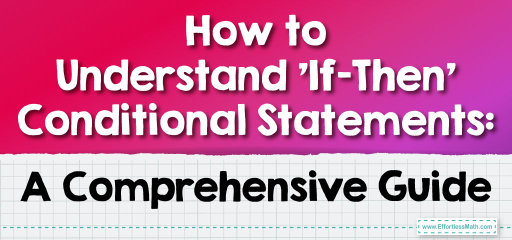# How to Understand ‘If-Then’ Conditional Statements: A Comprehensive Guide

In math, and even in everyday life, we often say 'if this, then that.' This is the essence of conditional statements. They set up a condition and then describe what happens if that condition is met. For instance, 'If it rains, then the ground gets wet.' These statements are foundational in math, helping us build logical arguments and solve problems. In this guide, we'll dive into the clear-cut world of conditional statements, breaking them down in both simple terms and their mathematical significance.## Step-by-step Guide: Conditional Statements

Defining Conditional Statements:
A conditional statement is a logical statement that has two parts: a hypothesis (the ‘if’ part) and a conclusion (the ‘then’ part). Written symbolically, it takes the form:
$$\text{If } p, \text{ then } q$$
Where $$p$$ is the hypothesis and $$q$$ is the conclusion.

Truth Values:
A conditional statement is either true or false. The only time a conditional statement is false is when the hypothesis is true, but the conclusion is false.

Converse, Inverse, and Contrapositive:
1. Converse: The converse of a conditional statement switches the hypothesis and the conclusion. For the statement “If $$p$$, then $$q$$”, the converse is “If $$q$$, then $$p$$”.

2. Inverse: The inverse of a conditional statement negates both the hypothesis and the conclusion. For the statement “If $$p$$, then $$q$$”, the inverse is “If not $$p$$, then not $$q$$”.

3. Contrapositive: The contrapositive of a conditional statement switches and negates both the hypothesis and the conclusion. For the statement “If $$p$$, then $$q$$”, the contrapositive is “If not $$q$$, then not $$p$$”.

### Examples

Example 1: Simple Conditional
Statement: “If it is raining, then the ground is wet.”

Solution:
Hypothesis $$( p )$$: It is raining.
Conclusion $$( q )$$: The ground is wet.

Example 2: Determining Truth Value
Statement: “If a shape has four sides, then it is a rectangle.”

Solution:
This statement is false because a shape with four sides could be a square, trapezoid, or other quadrilateral, not necessarily a rectangle.

Example 3: Converse, Inverse, and Contrapositive
Statement: “If a number is even, then it is divisible by $$2$$.”

Solution:
Converse: If a number is divisible by $$2$$, then it is even.
Inverse: If a number is not even, then it is not divisible by $$2$$.
Contrapositive: If a number is not divisible by $$2$$, then it is not even.

### Practice Questions:

1. Write the converse, inverse, and contrapositive for the statement: “If a bird is a penguin, then it cannot fly.”
2. Determine the truth value of the statement: “If a shape has three sides, then it is a triangle.”
3. For the statement “If an animal is a cat, then it is a mammal,” which of the following is its converse?
a) If an animal is a mammal, then it is a cat.
b) If an animal is not a cat, then it is not a mammal.
c) If an animal is not a mammal, then it is not a cat.

1. Converse: If a bird cannot fly, then it is a penguin.
Inverse: If a bird is not a penguin, then it can fly.
Contrapositive: If a bird can fly, then it is not a penguin.
2. The statement is true. A shape with three sides is defined as a triangle.
3. a) If an animal is a mammal, then it is a cat.

### What people say about "How to Understand ‘If-Then’ Conditional Statements: A Comprehensive Guide - Effortless Math: We Help Students Learn to LOVE Mathematics"?

No one replied yet.

X
30% OFF

Limited time only!

Save Over 30%

SAVE $5 It was$16.99 now it is \$11.99